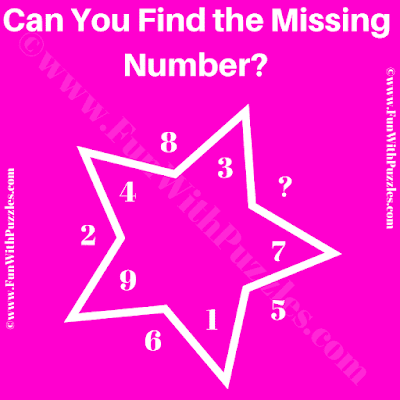This is a very simple math reasoning riddle for school students. This math riddle will test your mathematical skills and logical reasoning. In this reasoning riddle, you are shown some numbers in and around a Star. Your challenge is to find the logical or mathematical relationship among these numbers. Once you are able to decode the logic, find the value of the missing number which will replace the question mark.Can You Find the Missing Number?

The answer to this "Math Reasoning Riddle", can be viewed by clicking on the answer button.